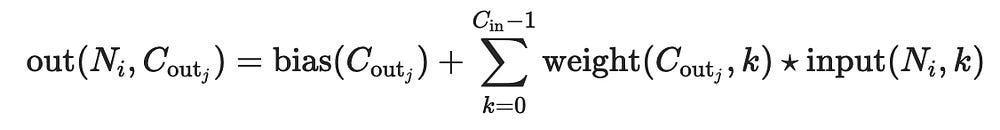# How groups work in PyTorch convolutions

Source: Deep Learning on Medium

### Part 1. Motivation

I have recently been working on a Deep Learning model implementation. I won’t talk much about details of the paper I was working on, at least for now. There is one thing I must mention to provide some context to this post. The paper proposed using a fixed number of weights per input channel. So, for example, we want to have 10 unique convolutional kernels. All ten of them we want to apply to each input channel. If the image has three input channels, then we apply all ten to the first channel, all ten to the second, and, finally, to the third.

I did not know how to properly approach this problem until I discovered groups in the PyTorch Conv2d documentation. So, after experimenting with this new parameter now I feel like this post might be useful for those who also face a similar task and want to get into understanding of Conv2d groups and utilizing the feature.

### Part 2. Notation

Let us agree on some notation tricks that helped me understand how groups work. I will link some visual representations as well, but for now, I want to focus on some pseudo-math.

(I say “pseudo” because it is not strictly mathematical to do what I am about to do, so for any fellow mathematicians out there, I apologize)

So, what does Conv2d do, really? Obviously, it computes a two-dimensional convolution, the operation without which there would be no point in writing this post. More specifically, as the docs put it:What Conv2d does under the hood (more or less). The star really denotes cross-correlation, but we won’t worry too much about that right now.

The value N_i here stands for the batch index. Let’s take a closer look at the summation part (I omit the bias term for now). It reminiscent of a certain mathematical operation, specifically, a matrix product. So, let’s denote:

For now we can view the Conv2d as a matrix operation, meaning that we will denoteNotation for the convolution in the Conv2d. We omit the bias term and let the batch size be 1 for simplicity.

### Part 3. Groups

If you look, by default the group parameter is set to 1. This means, according to the docs:

At groups=1, all inputs are convolved to all outputs.

That’s exactly what we saw in the formula above! Again, further in the docs we observe:

At groups=2, the operation becomes equivalent to having two conv layers side by side, each seeing half the input channels, and producing half the output channels, and both subsequently concatenated.

Let’s formalize this. First of all, we need to be able to split number of input channels in two, aka input channels must be an even number. Then, PyTorch creates weights W1 and W2 for each half of the new input. These groups of weights now output exactly half of the desired number of output channels, meaning that the number of channels must be divisible by 2. The weights take half the input channels. Then the formulas above are applied to each group (which now consists of two inputs and two sets of weights), the result of both convolutions is concatenated to produce the output of the desired shape.

So, for k groups in the setting of Conv2d, we must ensure that the number of input channels and the number of output channels are both divisible by k.

Say you want to take (1, 3, 64, 64) input image (tensor) and produce (1, 9, 64, 64) output tensor. You can have at most 3 groups (since 9mod3 = 0, 3mod3=0) in this situation and each channel will be convolved with 9/3=3 filters.

So, if you have (N, M, H, W) input shape where N is the batch size and M is the number of input channels, and you want to produce (N, L, H’, W’) output, the maximum number of groups you can have the Greatest Common Divisor of these two numbers.

### Part 4. Conclusion

Groups can be a useful feature, especially in models where each channel needs to be processed differently. If some input channels carry different information from others, then we might want to make the model learn to extract this relevant information channel-wise in the early layers. Hence, the groups parameter becomes really handy. It saves a great deal of time instead of processing each individual channel one by one.